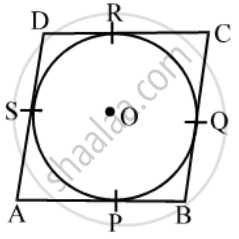# Prove that the Paralleogram Circumscribing a Circle, is a Rhombus - Mathematics

Prove that the paralleogram circumscribing a circle, is a rhombus

#### Solution

Given: A parallelogram ABCD circumsribes a circle with centre O.To prove: AB = BC = CD = AD

Proof: we know that the lengths of tangents drawn from an exterior point to a circle are equal.

∴ AP = AS …. (i) [tangents from A]

BP = BQ …. (ii) [tangents from B]

CR = CQ …. (iii) [tangents from C]

DR = DS …. (iv) [tangents from D]

∴ AB + CD = AP + BP + CR + DR

= AS + BQ + CQ + DS [From (i), (ii), (iii), (iv)]

= (AS + DS) + (BQ + CQ)

Hence, (AB + CD) = (AD + BC)

[∵ opposite sides of a parallelogram are equal]

∴ CD = AB = AD = BC

Hence, ABCD is a rhombus

Concept: Concept of Circle - Centre, Radius, Diameter, Arc, Sector, Chord, Segment, Semicircle, Circumference, Interior and Exterior, Concentric Circles
Is there an error in this question or solution?
Chapter 10: Circles - Exercise 10.2 [Page 214]

#### APPEARS IN

NCERT Class 10 Maths
Chapter 10 Circles
Exercise 10.2 | Q 11 | Page 214
Share